Select Page

# CBSE 12 Science Solutions for MCQ Maths Continuity and Differentiability in English

CBSE 12 Science Solutions for MCQ Maths Continuity and Differentiability in English to enable students to get Solutions in a narrative video format for the specific question.

Expert Teacher provides CBSE 12 Science Solutions for MCQ Maths Continuity and Differentiability through Video Solutions in English language. This video solution will be useful for students to understand how to write an answer in exam in order to score more marks. This teacher uses a narrative style for a question from Continuity and Differentiability not only to explain the proper method of answering question, but deriving right answer too.

Please find the question below and view the Solution in a narrative video format.

Question:

Solution Video in English:

You can select video Solutions from other languages also. Please check Solutions in ( Hindi )

## Similar Questions from CBSE, 12th Science, Maths, Continuity and Differentiability

Question 1 : Find the value of k, if the area of the triangle is 4 sq unit and vertices are (-2, 0), (0, 4), (0, k). (View Answer Video)

Question 2 : Differentiate the function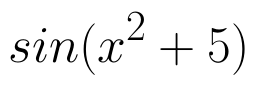with respect to x. (View Answer Video)

Question 3 : Differentiate the function w.r.t.x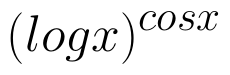. (View Answer Video)

Question 4 : Differentiate w.r.t.x the function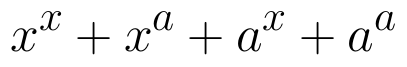, for some fixed a > 0 and x > 0. (View Answer Video)

Question 5 : If x and y are connected parametrically by the equation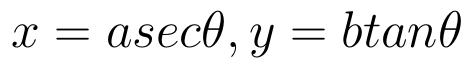, without eliminating the parameter, find. (View Answer Video)

### Differential Equations

Question 1 : Find the particular solution of the differential equation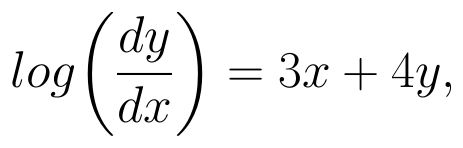given that y = 0, when x = 0. (View Answer Video)

Question 2 : Find the particular solution of the differential equation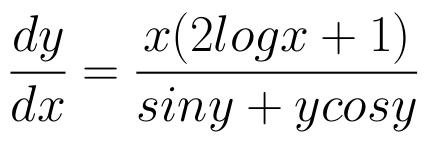given that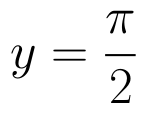where x = 1. (View Answer Video)

Question 3 : Find the sum of the order and the degree of the following differential equation: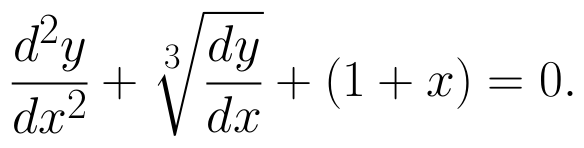(View Answer Video)

Question 4 : Solve the differential equation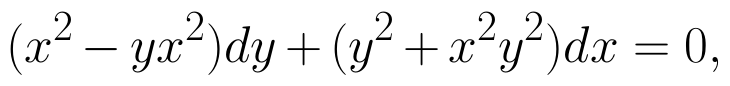given that y = 1 when x = 1. (View Answer Video)

Question 5 : Find the general solution of the following differential equation :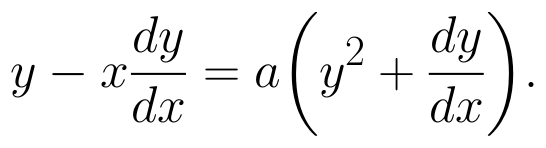(View Answer Video)

### Inverse Trigonometric Functions

Question 1 :

Write the value of the following: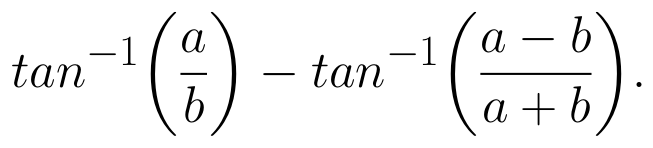Question 2 : Solve for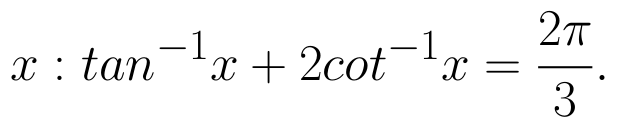(View Answer Video)

Question 3 : Write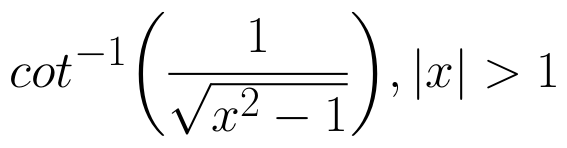in the simplest form. (View Answer Video)

Question 4 :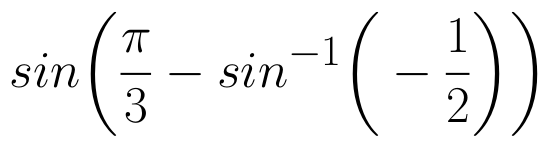is equal to :

Question 5 : Write in the simplest form: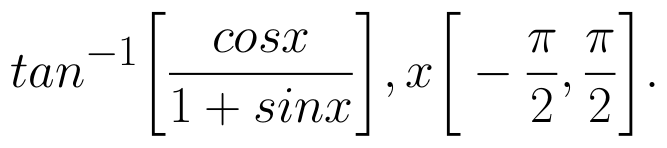(View Answer Video)

### Application of Derivatives

Question 1 : The slope of the normal to the curve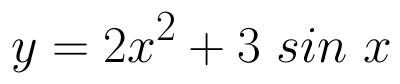at x = 0 is: (View Answer Video)

Question 2 : Find the approximate change in the surface area of a cube of side x meters caused by decreasing the side by 1%. (View Answer Video)

Question 3 : The function f is a differentiable function and satisfies the functional equation f(x) + f(y) = f(x + y) – xy – 1for every pair x, y of real numbers. If f(1) = 1, then the number of integers n ≠ 1 for which f(n) = n is_______________. (View Answer Video)

Question 4 : Find two positive numbers x  and y such that x+y=60 and is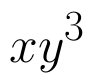maximum. (View Answer Video)

Question 5 : It is given that  at x=1, the function attains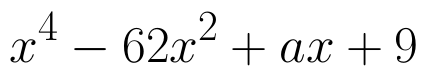its maximum value on the interval[0,2]. Find the value of a? (View Answer Video)